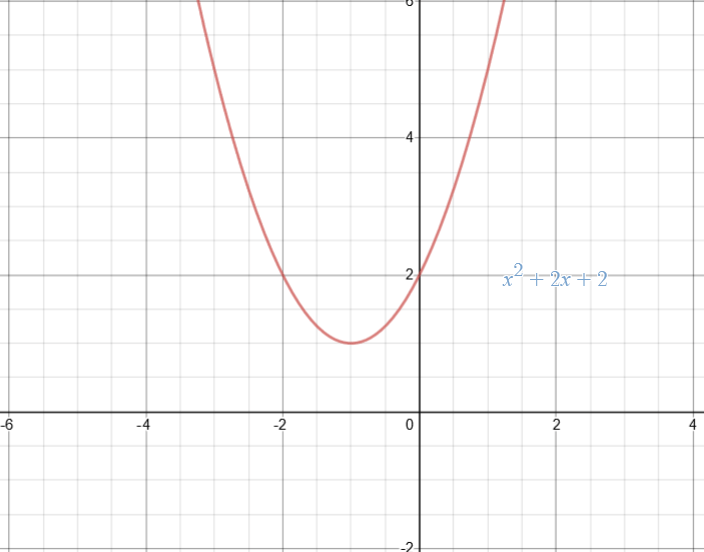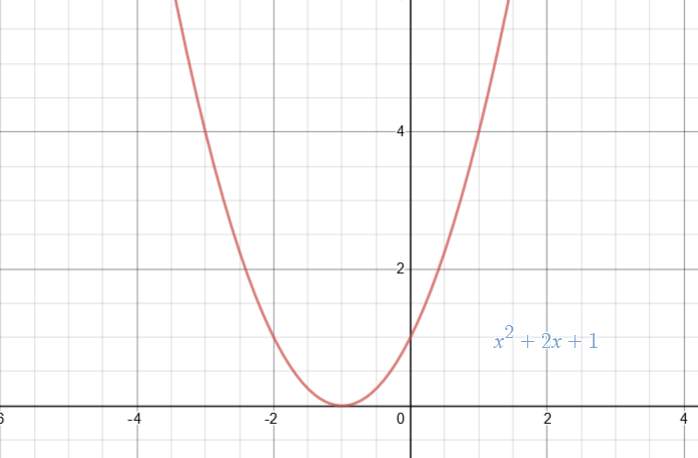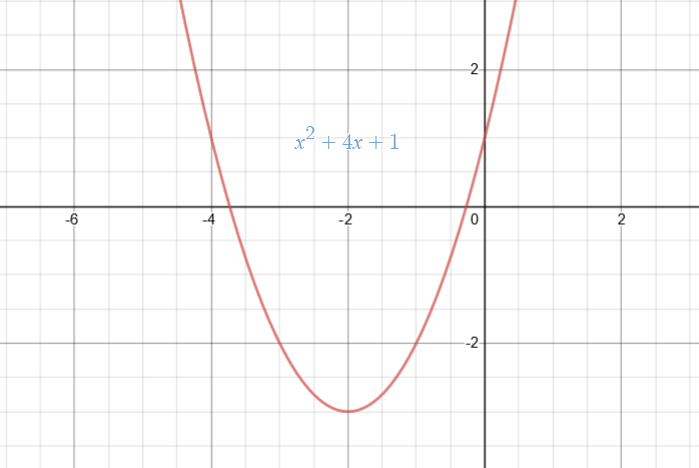# Discriminants

Contents

## Polynomial

You must have heard the word ‘polynomial’ or a ‘polynomial equation’. Algebra is obsessed with polynomials. You will find them everywhere. So, what is a polynomial then?

Polynomial is just an expression that involves variables and constants with arithmetic operators (‘+’, ‘-‘, ‘×’, ‘÷’) in between. An example of a polynomial is,$3x+4y^2+2$

As you can see that there are two variables, x and y, two coefficients i.e., 3 & 4 and a constant 2. Note that a constant is a number on its own, whereas a coefficient is a number multiplied with a variable. A polynomial can have any number of variables.

A polynomial can be as simple as, x, i.e., single variable polynomial with a coefficient of 1. Since polynomials involve variables, they can be written as functions of those variables. For example, we have the following single-variable polynomial (there is a single variable  x)$2x^3+3x-1$

You can see that it can also be written as the function of  as,$f(x)=2x^3+3x-1$

Therefore, polynomials can be written as functions and are therefore called the polynomial functions. Though, you will find the polynomial of a single variable represented as$P_n (x)$ in textbooks. The subscript ‘n’ represents the order of the polynomial$P_n (x)$.

The order/degree of a polynomial is the highest power of the variable in the polynomial expression. As an exercise, find the order of following polynomials.

•$x^5+2$
•$3x+2+6x^3$

Polynomial equation of order 1 is called a monomial equation e.g.,$P_1 (x)=2x$. A polynomial equation of 2nd order is called binomial or quadratic equation e.g.,$P_2 (x)=x^2+5x+2$. A polynomial equation of order 3 is called a trinomial equation e.g.,$P_3 (x)=x^3+4$ and so on.

From now on we will talk about polynomial equation of one variable only in the rest of this article.

## Roots of a polynomial equation

You may realize that a polynomial equation (of any degree) can be evaluated at different values of input (i.e., at different values of variable). In this way, we can observe how the output of a polynomial equation varies with respect to the value of the variable. We can also graph a polynomial function.

Now that we have understood that a polynomial can be evaluated at different values of the variable, we ask the inverse question i.e., “what are the possible values of the variable for which the polynomial has a certain value?” Most of the times in maths, it is of importance to find for which values of the variable, the polynomial equation hits the value of 0.

Interesting fact: Graphically, the number of times a polynomial function hits 0 is less than or equal to the order of the polynomial function.

Roots of a polynomial equation are those values of the variable for which the polynomial becomes zero. In other words, the roots of a polynomial equation are the solutions of the equation,$a_n x^n+a_{n-1} x^{n-1}+...+a_1 x+a_0=0$

The number of roots depends on the degree of the polynomial. In fact, the number of roots of a polynomial equation is equal to the degree of that polynomial equation.

For example, the polynomial equation$x^2-4=0$ has two roots i.e.,$\pm 2$. If you plug in$x=\pm 2$ in the given equation, you will get 0. Note that the degree of polynomial is 2, same as the number of roots.

You may, however, realize that the equation,$x^2+1=1$, has only one root i.e., 0, even though the degree of the polynomial is 2. Mathematicians interpret this as a case of repeating roots. In other words, there are 2 roots of this equation, both equal to 0.

Finding roots of complex equations is a topic of research in maths. For polynomial equations, we define a term called Discriminant that helps in finding the roots of the equations.

### Discriminant

It is a special kind of quantity that is evaluated with help of unique mathematical relations between the coefficients and constants of a polynomial equation. The discriminant helps determine different characteristics or features of the roots of a polynomial equation. Sometimes knowing the nature of roots is the only information that we seek. Having awareness of the nature of roots helps in deciding whether to proceed with the solution or not. For instance, if we are looking for the equations (and their solutions) that have a particular property, we can know the nature of roots before even solving the equations and based on that information we can decide whether we would like to invest our time in finding the solutions or not.

In this article, we will use the word ‘Discriminant’ to refer to the discriminant of the quadratic equation only.

We can write a general quadratic (2nd order polynomial) equation as,$f(x)=ax^2+bx+c$

You can think of any example of quadratic equation and will find that indeed this is a general representation of the quadratic equation. Now, we would like to find the roots of the equation,$ax^2+bx+c=0$

In other words, we would like to find the values of x that satisfy the above equation. You can recall (or if you do not know already, you can find the derivation in literature) that the roots of above equation are given by the famous quadratic formula,$x_{1,2}= \frac{-b\pm \sqrt{b^2-4ac}}{2a}$

It is important to note that$x_{1,2}$ represents two roots$x_{1}$ and$x_{2}$. Both$x_{1}$ and$x_{2}$ are constants (not variables!).

Let us solve an example to see the quadratic formula in action. We apply the quadratic formula to find the roots of the equation$x^2+5x+4=0$.

The first step in finding the roots is to compare the given equation with the standard quadratic equation and extract the values of coefficients. After comparing the two equations, we find that,

• a = 1
• b = 5
• c = 4

Now, we put the above coefficients in the quadratic formula. The roots of the given equation come out to be,$x_1 = \frac{-b+\sqrt{b^2-4ac}}{2a}$$x_1 = \frac{-5+\sqrt{25-16}}{2}=-1$

And,$x_2 = \frac{-b-\sqrt{b^2-4ac}}{2a}$$x_2 = \frac{-5-\sqrt{25-16}}{2}=-4$

Therefore, the two roots of the equation$x^2+5x+4=0$ come out to be -1 and -4. It is also important to note that we can construct the original quadratic equation with the information of roots. This is done by representing the roots as two individual first order equations and taking their product.$x=-1$$x+1=0$

Similarly,$x=-4$$x+4=0$

Now taking the product,$(x+1)(x+4)=0$$x^2+5x+4=0$

That is how we get the original equation.

Exercise: As an exercise, find the roots of the following equations.

•$x^2+3x=0$
•$4x^2=10x+3$ (Hint: Convert it into standard form first)

The students are expected to memorize this quadratic formula as it is extensively used everywhere in maths, physics and engineering fields. You will make use of the quadratic formula frequently while solving different types of equations.

## Using discriminant to find the nature of the roots of quadratic equation

The expression$(b^2-4ac)$ under the square root of the quadratic formula,$x_{1,2}= \frac{-b\pm \sqrt{b^2-4ac}}{2a}$, is known as the discriminant of the quadratic equation.

The discriminant is used to find the nature of the roots. Depending on the relative magnitudes of the coefficient, three cases occur.

1.$b^2-4ac<0$
2.$b^2-4ac=0$
3.$b^2-4ac>0$

Let us explore the three cases in detail.

## Case-I (Discriminant < 0)

The first case occurs when the value of discriminant is less than 0 i.e., the discriminant is negative. It can be observed that if the discriminant is negative, the quadratic formula becomes.$x_{1,2}= \frac{-b\pm \sqrt{-k^2}}{2a}$

where,$k^2=|b^2-4ac|$ (the positive absolute value of the discriminant)

Note that$k^2$ is a positive number. Thus, we have$x_{1,2}= \frac{(-b\pm \sqrt{-1})}{2a} = \frac{(-b\pm ki)}{2a}$

In other words, the roots happen to be complex when discriminant < 0. Also, the roots are distinct and appear as conjugate pairs. The condition$b^2-4ac<0$ can be further simplified as$b^2<4ac$.

Now we plot the function$x^2+2x+2$ (Observe that$b^2=2^2=4$ and$4ac=8$, i.e.,$b^2-4ac<0$.The graph presents some interesting facts. It can be seen that the function gets to a minimum value of 1, and the curve does not intersect y = 0 line at a single point. In other words, there is not a single real value of x for which the function$x^2+2x+2$ is 0 i.e., no real solution exists. This is the reason that we get complex roots for the equation$x^2+2x+2=0$. It should be noted that complex values always appear as complex conjugate pairs. Now you must be able to even tell the nature of roots by looking at the graph of a quadratic equation or, conversely, tell that whether the curve crosses the line y = 0 (i.e., x-axis) by knowing the nature of the roots.

Caution: Do not mix up the two expressions:

•$ax^2+bx+c$
•$ax^2+bx+c=0$

The first expression represents a function that can be plotted to get a graph. It gets different x values as input and returns$(ax^2+bx+c)$ as output. On the other hand,$ax^2+bx+c=0$ is an equation. Its purpose is to find the value of x for which$ax^2+bx+c$ is 0. The roots are found for$ax^2+bx+c=0$ (not just$ax^2+bx+c$). In other words, to say that “find roots of$ax^2+bx+c$ makes no sense.

## Case-II (Discriminant = 0)

The second case occurs when the value of discriminant is equal to 0. It can be observed that when the discriminant is 0, the quadratic formula simplifies as,$x_{1,2}= \frac{-b\pm \sqrt{0}}{2a}$$x_{1,2}= \frac{-b}{2a}$

Thus, the roots of the quadratic equation are real and equal when discriminant is 0. In other words, we can say that the roots are real and repeated. The condition$b^2-4ac=0$ can be simplified as$b^2=4ac$. It can be reiterated in other words as the roots of a quadratic equation happen to be real and repeated when$b^2=4ac$.

Now we plot a relevant function$x^2+2x+1$ (Observe that$b^2=2^2=4$ and$4ac=4$ i.e.,$b^2=4ac$).Finally, we see something real (real roots). Again, useful information can be extracted from such a graph. Notice that the curve crosses the x-axis at only one point i.e., x = -1. Thus, we can conclude that the roots of the equation$x^2+2x+1=0$ are real (because of the fact that the curve did touch/cross the x-axis and roots are repeated (because of the fact that the x-axis was crossed at only one point).

## Case-III (Discriminant > 0)

The third case occurs when discriminant > 0 i.e., when the discriminant is positive. In this case, the quadratic equation simplifies as$x_{1,2}= \frac{(-b\pm \sqrt{-k^2})}{2a}$

where,$k^2=|b^2-4ac|$ (the positive absolute value of the discriminant)

Note that$k^2$ is a positive number. Thus, we have$x_{1,2}= \frac{(-b\pm k)}{2a} = \frac{(-b\pm k)}{2a}$

In short, the roots are real and distinct when the discriminant is positive. The condition$b^2-4ac>0$ can be further simplified as$b^2>4ac$. Therefore, the roots of a quadratic equation are real and distinct when$b^2>4ac$.It is noted that the curve crosses x-axis at two different locations. In other words, the roots of the equation$x^2+4x+1=0$ are real (because the real x-axis is crossed by the curve) and distinct (because x-axis is crossed by the curve at two different values of x).

We can conclude the three cases as:

1.$b^2-4ac<0$ (Roots are complex and distinct)
2.$b^2-4ac=0$ (Roots are real and repeated)
3.$b^2-4ac>0$ (Roots are real and distinct)

Exercise: Apply the concept of discriminant to find the nature of the roots of the following equations.

•$x^2+5x+3=0$
•$2x^2-4x-1=0$
•$x^2+2x=3$

Bonus Exercise: It is known that the roots of a polynomial equation$px^2+qx+r=0$ are real and repeated. Based on this information, find the nature of the roots of the following equations.

•$2px^2+qx+r=0$
•$px^2+4qx+r=0$
•$8px^2+4qx+2r=0$